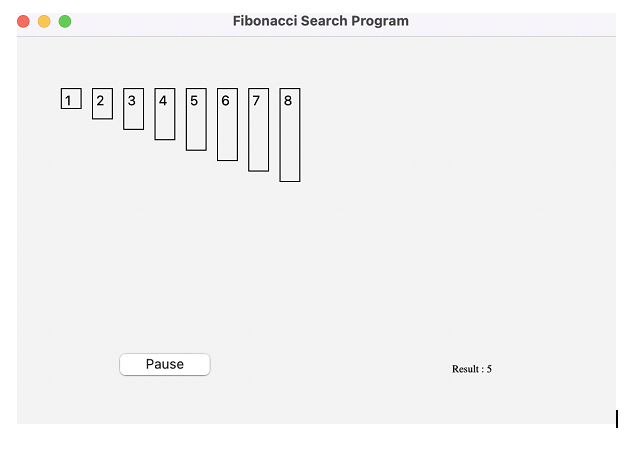# Fibonacci Search Visualizer using PyQt5

Sorting of lists help us in solving sort huge amount data and various mathematical and logical problems in a very less time. We can find a particular element in a sorted list easily with the help of Fibonacci search method. Here, we will create a Fibonacci Search Visualiser using a PyQt5 module in Python.

## Example

In this example, we have used a Fibonacci visualizer's user interface that consists of a window with a list of fibonacci numbers and display the result.

The following PyQt5 widgets are used in this code:

QListWidget

QLineEdit

QPushButton

### Algorithm

Step-1: Take the list of numbers and the element to be searched is provided as .

Step-2: Find the two closest Fibonacci numbers that are greater than or equal to the length of the list.

Step-3: Initialize the low and high indices with 0 and first number in Fibonacci list.

Step-4: Repeat the following steps while the element is not found and the length of the search interval is greater than 1: Calculate the index of the middle element. Calculate the index of the middle element in the second part using the first and second Fibonacci numbers, Compare the elements, if the element is less than the middle element, search in the first part, update the high index and Fibonacci numbers accordingly.

Step- 5: If the element is greater than the middle element, search in the second part and If the element is equal to the middle element, return the index of the middle element.

# importing libraries
from PyQt5.QtWidgets import *
from PyQt5 import QtCore, QtGui
from PyQt5.QtGui import *
from PyQt5.QtCore import *
import sys

class SearchWindow(QMainWindow):
number = [1, 2, 3, 4, 5, 6, 7, 8]
desired = 5

def __init__(windo):
super().__init__()
windo.setWindowTitle("Fibonacci Search Program")
windo.setGeometry(100, 100, 600, 400)
windo.UiComponents()
windo.show()

def UiComponents(windo):
windo.start = False
windo.divide = False
windo.fib_search = True
windo.label_list = []
windo.fib1 = 1
windo.fib2 = 0
windo.fib = windo.fib1 + windo.fib2

windo.offset = -1

# local counter
count = 0

for i in windo.number:

label = QLabel(str(i), windo)

label.setStyleSheet("border : 1px solid black;")
label.setAlignment(Qt.AlignTop)
label.setGeometry(50 + count * 30, 50, 20, i * 10 + 10)
windo.label_list.append(label)

count = count + 1

windo.search_button = QPushButton("Start Search", windo)

windo.search_button.setGeometry(100, 300, 100, 30)

windo.search_button.clicked.connect(windo.search_action)

pause_button = QPushButton("Pause", windo)

pause_button.setGeometry(100, 300, 100, 30)

# adding action to the search button
pause_button.clicked.connect(windo.pause_action)

# creating label to show the result
windo.result = QLabel("Result : " + str(windo.desired), windo)

windo.result.setGeometry(320, 300, 250, 40)

windo.result.setStyleSheet("border : solid black;")
windo.result.setFont(QFont('Times', 10))

windo.result.setAlignment(Qt.AlignCenter)

timer = QTimer(windo)

timer.timeout.connect(windo.showTime)

timer.start(300)

def showTime(windo):

# checking if flag is true
if windo.start:

if windo.fib_search:

if windo.fib < len(windo.number):

windo.fib2 = windo.fib1
windo.fib1 = windo.fib
windo.fib = windo.fib2 + windo.fib1
windo.result.setText("Searching Fibonacci number >=" +
str(windo.desired))
else:
windo.result.setText("Fibonacci found, searching number")
windo.fib_search = False
windo.divide = True

if windo.divide:

if windo.fib <= 1:
windo.result.setText("Number not available")
windo.start = False
return

i = min(windo.offset + windo.fib2, len(windo.number) - 1)

windo.label_list[i].setStyleSheet("border : 1px solid black;"
"background-color : grey")

if (windo.number[i] < windo.desired):
windo.fib = windo.fib1
windo.fib1 = windo.fib2
windo.fib2 = windo.fib - windo.fib1
windo.offset = i
elif (windo.number[i] > windo.desired):
windo.fib = windo.fib2
windo.fib1 = windo.fib1 - windo.fib2
windo.fib2 = windo.fib - windo.fib1

else:
windo.result.setText("Found at : " + str(i))
windo.label_list[i].setStyleSheet(
"border : 2px solid green;"
"background-color : lightgreen;")
windo.start = False

def search_action(windo):

windo.start = True
windo.result.setText("Started searching...")
def pause_action(windo):

windo.start = False
windo.result.setText("Paused")
App = QApplication(sys.argv)
window = SearchWindow()
sys.exit(App.exec())


### OutputIn using the Fibonacci search algorithm, we identify the two Fibonacci numbers that are most closely related to the length of the list being searched. After dividing the list into three sections using these two values, we can compare the element we're looking for to the middle element in each section. Depending on the results of the comparison, we either continue looking in the first or second portion of the list until we discover the element or come to the conclusion that it doesn't exist.

## Conclusion

The Fibonacci Search Visualizer we developed using PyQt5 enables us to see how the Fibonacci search algorithm functions. We may input a search term and watch how the algorithm splits the list into three sections and does the search. The visualizer can be helpful for troubleshooting and comprehending the method.

Updated on: 10-Aug-2023

71 Views